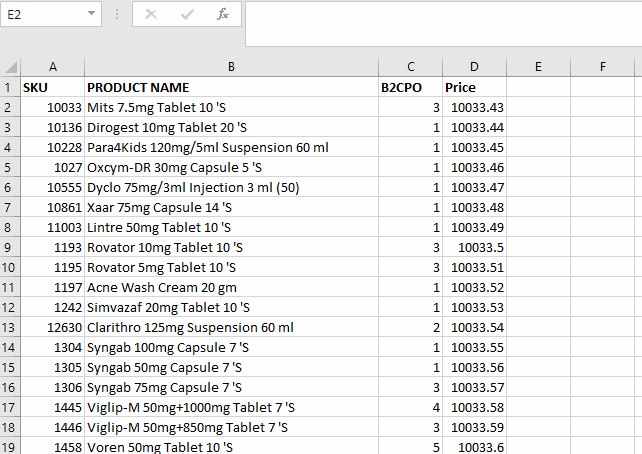# How to round to nearest whole number in Excel

This guide will help you round any number to the nearest whole number in Excel. Microsoft Excel provides a built-in function of round off. The formula is given below;

=ROUND(value,0)

In the formula phrase, select the desired value  then we can set the integer value where we wish to round off.Microsoft Excel is a very powerful tool for numeric data computation and calculation. Microsoft Excel offers a variety of functions and formulas that can help you round numbers up or down.

### Step 1 – Select the data range and apply the formula